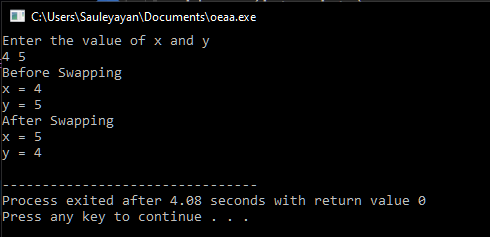Open in App
Not now

# C++ Functions – Pass By Reference

• Last Updated : 05 Nov, 2022

Several ways exist in which data (or variables) could be sent as an argument to a function. Two of the common ones are Passing by Value and Passing by Reference. Passing by reference allows a function to modify a variable without creating a copy. We have to declare reference variables. The memory location of the passed variable and parameter is the same. Therefore, any change to the parameter also reflects in the variable inside its parent function. This article focuses on discussing how to pass variables by reference in C++.

## What is a Pass by Reference?

When a variable is passed as a reference to a function, the address of the variable is stored in a pointer variable inside the function. Hence, the variable inside the function is an alias for the passed variable. Therefore, any operations performed on the variable inside the function will also be reflected in the calling function.

• This ability to reflect changes could return more than one value by a function.
• Also, a void function could technically return value/s using this method.

The & (address of) operator denotes values passed by pass-by-reference in a function. Below is the C++ program to implement pass-by-reference:

## C++

 `// C++ program to implement ``// pass-by-reference``#include ``using` `namespace` `std;`` ` `void` `f(``int` `& x) ``{``  ``x--;``}`` ` `// Driver code``int` `main() ``{``  ``int` `a = 5;``  ``cout << a << endl;``  ``f(a);``  ``cout << a << endl;``}`

Output

```5
4```

Explanation:

• Firstly a function is defined with the return datatype void and takes in value by reference (as denoted by the & address sign in the formal parameters).
• The function decrements the value of its formal parameter by 1.
• After which, inside the main function, an integer variable named a is initialized with the value 5.
• The value of ‘a’ is printed on the console. The f() function is called, and the variable is passed as an argument.
• Inside the function, the variable’s value is decremented by 1.
• Upon returning from the function, the variable’s value is again displayed, which turned out to be 1 less than the original value.

Hence, the changes to a variable passed by reference to a function are reflected in the calling function.

## Swap function using Pass-By-Reference

The swap function swaps the values of the two variables it receives as arguments. Below is the C++ program to swap the values of two variables using pass-by-reference.

## C++

 `// C++ program to swap the values``// of two variables using ``// pass-by-reference``#include `` ` `// Prototype of the function``void` `swap(``int` `&, ``int` `&);``  ` `// Driver code``int` `main()``{``  ``int` `x, y;``  ` `  ``// Inputting two variables``  ``printf``(``"Enter the value of x and y\n"``);``  ``scanf``(``"%d%d"``, &x, &y);``  ` `  ``// Displaying their values before swapping``  ``printf``(``"Before Swapping\nx = %d\ny = %d\n"``, ``          ``x, y);``  ` `  ``// Calling the function & sending variable ``  ``// values as argument      ``  ``swap(x, y); ``  ` `  ``// Displaying their values after swapping``  ``printf``(``"After Swapping\nx = %d\ny = %d\n"``, ``          ``x, y);``  ``return` `0;``}``  ` `// Function uses pass by reference method ``// to swap passed variable values``void` `swap(``int` `&a, ``int` `&b)``{``  ``int` `temp;``  ` `  ``temp = b;``  ``b = a;``  ``a = temp;   ``}`

Output:Explanation:

• Firstly the function prototype is defined (optional if the function is defined before the main function).
• Inside the main function, the values of the two variables are taken as input from the user.
• The values before calling the swap function are printed on the console.
• After which, the values are passed as an argument to the swap function.
• The swap function utilizes call-by-reference and contains the code for swapping the two variables.
• Upon completion of the function, the value of the two variables is displayed in the main function (after the call to swap).
• The swapped values are displayed on the screen.

## Pass by Reference with Pointers

It is also possible to pass the variable address from the calling function and use them as a pointer inside the called function. This allows a bit more explicitly in the change of variable values in the function.

Below is the C++ program to implement pass-by-reference with pointers:

## C++

 `// C++ program to implement``// pass-by-reference with``// pointers``#include ``using` `namespace` `std;`` ` `void` `f(``int` `*x) ``{``  ``*x = *x - 1;``}`` ` `// Driver code``int` `main() ``{``  ``int` `a = 5;``  ``cout << a << endl;``  ``f(&a);``  ``cout << a << endl;``}`

Output

```5
4```

Explanation:

• Firstly a function is defined with the return datatype void and takes in value as pointers (as denoted by the * asterisk sign in the formal parameters).
• The function decrements the value of its formal parameter by 1.
• After which, inside the main function, an integer variable named ‘a’ is initialized with the value 5.
• Then this value is displayed. The function is called, and the address of the variable is passed as an argument.
• Inside the function, the pointer variable’s value is decremented by 1.
• Upon returning from the function, the variable’s value is again displayed, which turned out to be 1 less than the original value.

My Personal Notes arrow_drop_up